# HOTS: Higher-Order Thinking Skills

Develop critical thinking, connect math concepts and master problem solving with the toughest questions available in KooBits.
Loved by 200,000+ parents & students with proven results!

## Why use HOTS?

### Go beyond facts and memorisation

Effective way to expand your child’s thinking

### Build a high-demand skill

Transform your child into a lifelong learner, and prepare them for the real world

### Create a sense of achievement

HOTS questions aren’t easy. But doing them will boost your child’s motivation in math

Higher-Order Thinking Skills (HOTS) distinguishes critical thinking skills from low-order learning outcomes, such as those attained by rote memorization.

HOTS is based on various taxonomies of learning, particularly by Benjamin Bloom in 1956. The chart shows a revised version of Bloom’s taxonomy from Anderson, L. W. & Krathwohl, D.R., et al (2001).

HOTS is believed to better prepare children beyond the standard school maths curriculum, and empower them to interpret ideas, information, discover new solutions and improve their understanding in maths.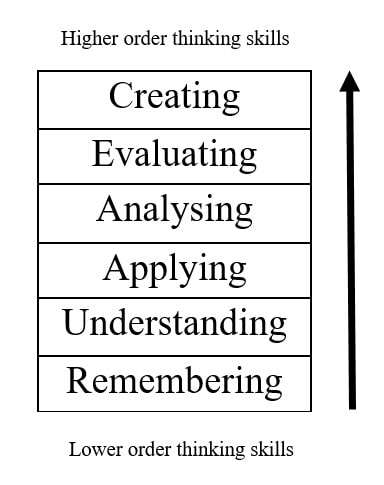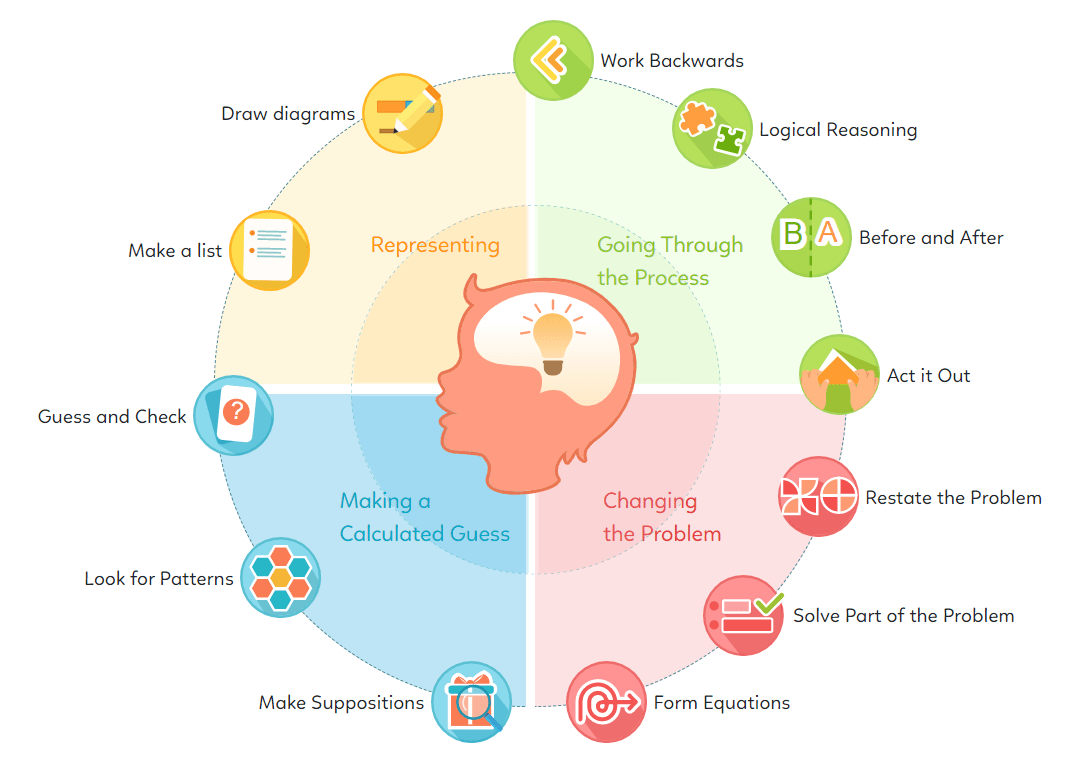Students who have good level of HOTS are expected to succeed in their studies later."
With HOTS, your child is likely to achieve:

## Let's see how HOTS makes difference

### Standard Question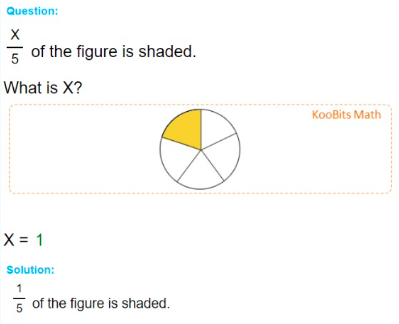### HOTS Question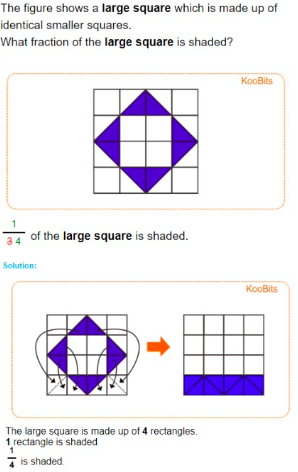HOTS questions encourage your child to explore, interpret ideas and arrive at solutions by themselves.

Every topic at every grade level is specially designed to improve problem solving skills which can then be applied to real life situations.

• Level 1
• Level 2
• Level 3
• Level 4
• Level 5
• Level 6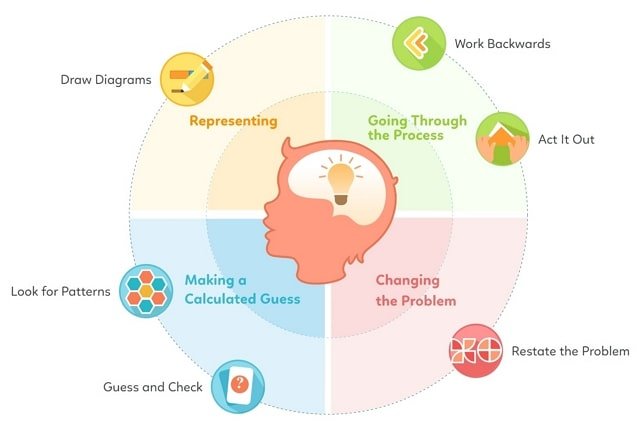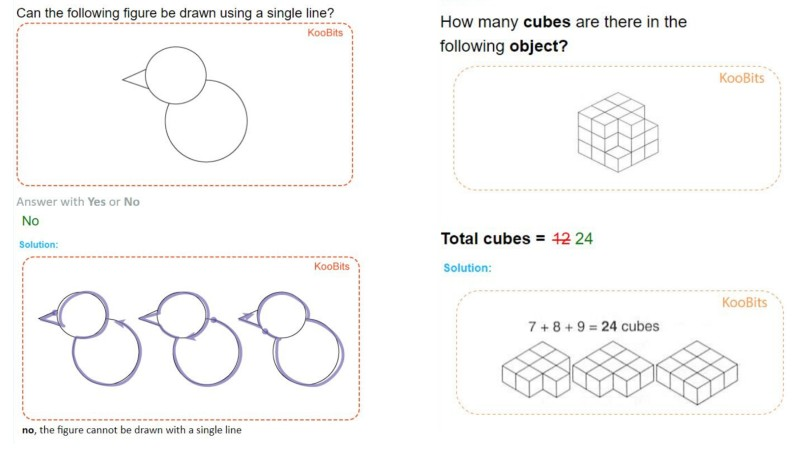Draw Diagrams
• Whole Numbers - Buy this and Get that Free
• Length - Points along a Straight Line
• Length - Finding Two Unknown Values
• Mass - Finding Two Unknown Values
• Money - Finding Two Unknown Values
• Money - Spending Money
• Measurement - Time
Guess and Check
• Whole Numbers - Pictorial Questions
• Whole Numbers - Finding Two Unknown Numbers
• Measurement - Finding Two Unknown Numbers
Look for Patterns
• Whole Numbers - Finding Missing Number in a Sequence
• 2D Shapes - Finding Patterns
• 2D Shapes - Stacking Up
• 3D Shapes - Stacking Up
Restate the Problem
• Whole Numbers - Subtraction
• Whole Numbers - Addition and Subtraction (Mixed)
Work Backwards
• Whole Numbers - Multiplication & Division
• Mass - Give and Share
• Money - Give and Share
Act it Out
• Geometry - Drawing with One Line
• Geometry - Arranging on the Sides
• Whole Numbers - Number of ways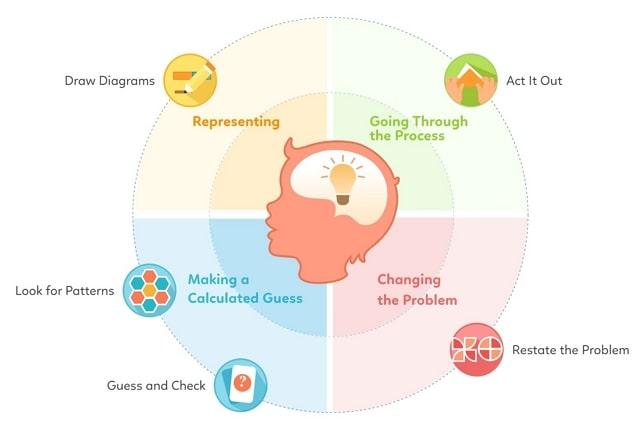Draw Diagrams
• Whole Numbers - Give and Take Questions
• Whole Numbers - Sum and Difference Questions
• Whole Numbers - Division using Multiplication Table of 2, 5, 10, 3, 4
• Fractions - Comparing Fractions
• Fractions - Word Problems involving Addition and Subtraction of Fractions
• Length - Word Problems involving Addition and Subtraction
• Volume - Word Problems involving Addition and Subtraction
• Mass - Word Problems involving Addition and Subtraction
• Money - Word Problems involving Addition and Subtraction
• Time - Word Problems involving Addition and Subtraction
Guess and Check
• Whole Numbers - Rabbit and Chicken Questions
• Whole Numbers - Numbers in a Magic Square
• Money - Shopping
Look for Patterns
• Whole Numbers - Addition and Subtraction
• Whole Numbers - Four Operations
• 2D Shapes
Restate the Problem
• Whole Numbers - Addition involving two numbers up to 3 digits
• Whole Numbers - Subtraction involving two numbers up to 3 digits
• Whole Numbers - Multiplication Table of 2, 5, 10, 3, 4
Logical Reasoning
• Whole Numbers - Number Positions
• Whole Numbers - Number Story
Act it Out
• Fractions - Shaded Parts of a Square
• Whole Numbers - Number Positions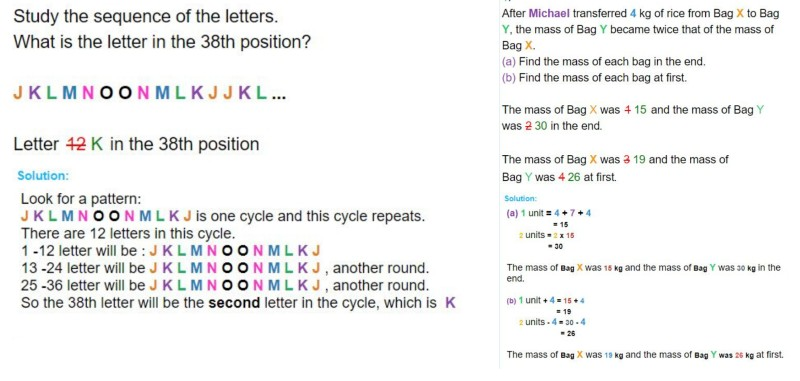Draw Diagrams
• Whole Numbers - Word Problems Involving Multiplication and Division
• Fractions - Simplest Form and Equivalent Fractions
• Fractions - Addition and Subtraction of Fractions
Look for Patterns
• Whole Numbers - Number Patterns
• Whole Numbers - Number Sequence and Forming Patterns From Diagrams
• Whole Numbers - Number Patterns in a Row
Restate the Problem
• Whole Numbers - Addition and Subtraction
• Whole Numbers - Multiplication
Work Backwards
• Whole Numbers - Four Operations
• Length - Finding Origin
• Volume - Finding Origin
• Mass - Finding Origin
• Money - Finding Origin
Before and After
• Length - Before and After
• Mass - Before and After
• Money - Before and After
Act it Out
• Perpendicular and parallel lines
• Area and PerimeterDraw Diagrams
• Whole Numbers - Missing Numbers
• Whole Numbers - Factors and Multiples
• Fractions - Part-Whole Relationship
• Decimals - Word Problems Involving Decimals
• Measurements - Finding the Starting Value
Make a List
• Whole Numbers - Factors and Multiples
• Whole Numbers - Finding the Number of Ways
• Area and Perimeter of Squares and Rectangles
Guess and Check
• Whole Numbers - Missing Numbers
• Money - Word Problems involving Money
• Mass - Word Problems involving mass
Make Suppositions
• Whole Numbers - Word Problems
Restate the Problem
• Whole Numbers - Four Operations
• Whole Numbers - Missing Numbers
• Fractions - Part-Whole Relationship
• Fractions - Comparing Fractions
Solve Part of the Problem
• Measurements - Finding the Starting Value
• Area and Perimeter of Squares and Rectangles
Logical Reasoning
• Length - Tree Planting
• Symmetry
Act it Out
• Symmetry
• Tessellation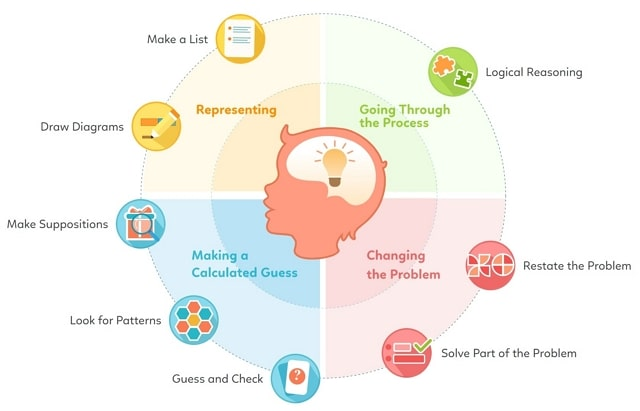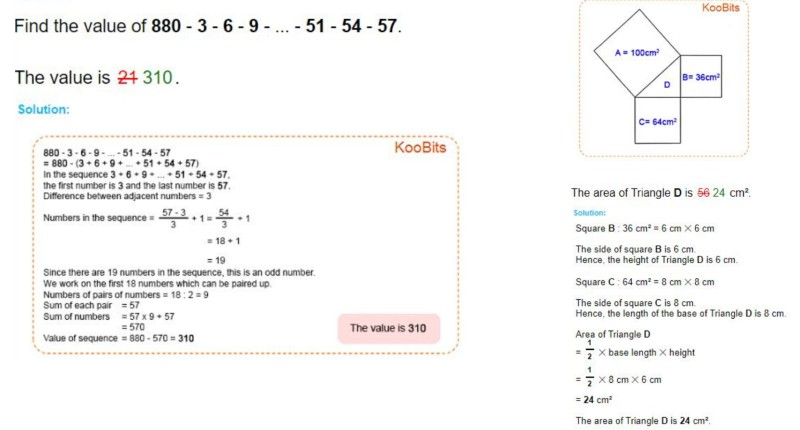Draw Diagrams
• Whole Numbers - Dividend, Quotient, Divider and Remainder
• Whole Numbers - Finding Unknown Numbers
• Whole Numbers - The Question of Age
• Fractions - Part-Whole Concept (Word Problem)
• Fractions - Comparison Concept (Word Problem)
• Fractions - Equal Concept (Word Problem)
• Fractions - Remainder Concept (Word Problem)
• Decimals - Word Problems Involving Decimals
• Decimals - Comparison Concept (Word Problem)
• Percentage - Part-Whole Relationships
• Ratio - Part-Whole Relationships
• Measurement - Replacement and Elimination Questions
Make a List
• Whole Numbers - Dividend, Quotient, Divider and Remainder
• Money - Word Problems involving Money
Guess and Check
• Whole Numbers - Finding Unknown Numbers
• Whole Numbers - Counting Wheels
• Whole Numbers - The Question of Age
Look for Patterns
• Whole Numbers - Dividend, Quotient, Divider and Remainder
• Whole Numbers - Seat Number Problem
• Whole Numbers - Summation of Sequences
• 4-sided Figures and Number Sequence
Make Suppositions
• Whole Numbers - Finding Unknown Numbers
• Whole Numbers - Word Problems Involving Unitary Value
• Money - Word Problems Involving Unitary Value
Restate the Problem
• Whole Numbers - Finding Unknown Numbers
• Whole Numbers - Dividend, Quotient, Divider and Remainder
• Whole Numbers - Word Problems Involving Unitary Value
• Money - Word Problems Involving Unitary Value
• Money - Coin Change Problem
• Fractions - Comparing Fractions
• Fractions - Part-Whole Relationships
• Decimals - Repeated Variable Concept (Word Problems)
• Percentage - Part-Whole Relationships
• Ratio - Part-Whole Relationships
• Measurement - Replacement and Elimination Questions
• Area of Triangle
• Finding Angles
Solve Part of the Problem
• Area of Triangle
• Cubes and Cuboids
• Finding Angles
Before and After
• Whole Numbers - Quantity Comparison
• Whole Numbers - Age Problem
• Money - Find initial amount
• Fractions - Change Concept (Word Problems)
Logical Reasoning
• Whole Numbers - Dividend, Quotient, Divider and Remainder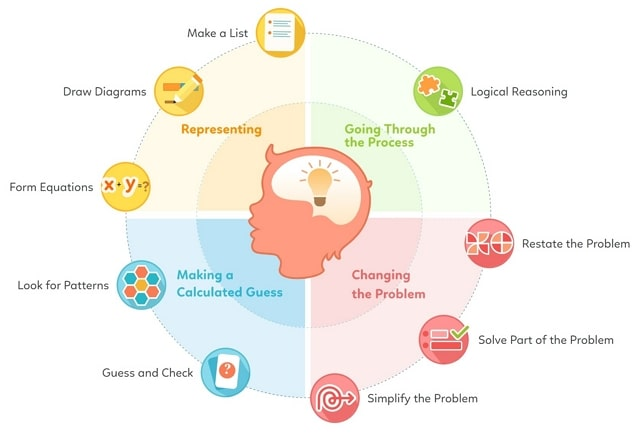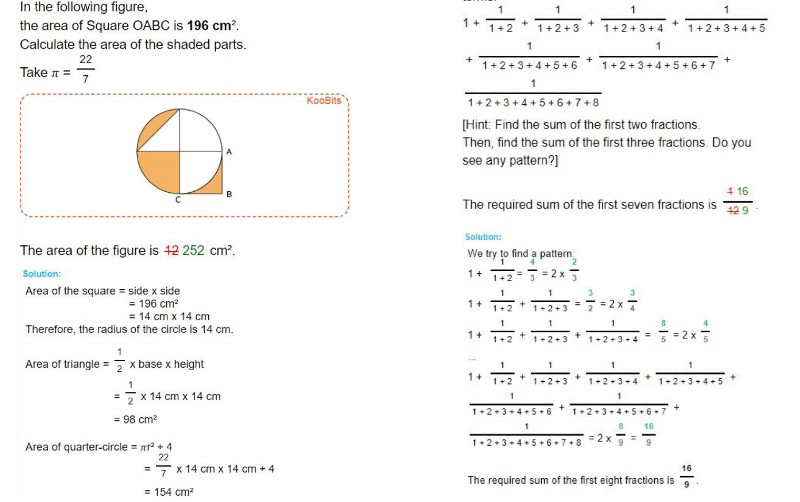Draw Diagrams
• Fractions - Word Problems involving Fractions
• Ratio - Word Problems Involving Ratio
• Percentage - Word Problems Involving Percentage
• Speed - Word Problems Involving Speed
• Measurements - Word Problems
• Algebra - Word Problems involving Algebra
Make a List
• Whole Numbers - Sharing Problems
• Area and Perimeter of Squares and Rectangles
• Area and Perimeter of Triangles
Form Equations
• Area and Perimeter of Circles and Composite Figures
• Algebra - Word Problems involving Algebra
Guess and Check
• Whole Numbers - Sharing Problems
• Fractions - Types of Fractions
• Measurements - Word Problems
Look for Patterns
• Number Patterns
• Fractions - Sum of fractions
Restate the Problem
• Decimals - Mental Calculation
• Whole Numbers - Sum Up Numbers
• Average
• Whole Numbers - Sharing Problems
• Ratio - Word Problems Involving Ratio
• Percentage - Word Problems Involving Percentage
• Speed - Word Problems Involving Speed
• Measurements - Word Problems
Simplify the Problem
• Algebra - Word Problems involving Algebra
Solve Part of the Problem
• Ratio - Word Problems Involving Ratio
• Percentage - Word Problems Involving Percentage
• Speed - Word Problems Involving Speed
• Measurements - Word Problems
• Area and Perimeter of Triangles, Squares and Rectangles
• Area and Perimeter of Circles and Composite Figures
• Volume of Cube and Cuboid
• Rate of Water Flow into Rectangular Tanks
• Algebra - Word Problems involving Algebra
Logical Reasoning
• Fractions - Types of Fractions

## The Maths Platform Children Love to Use

Where Learning Comes to Life

Higher-Order Thinking Skills

The Ultimate Challenge for Maths Mastery

Top Schools’ Questions in One Place

Support
Resources
Let's Connect